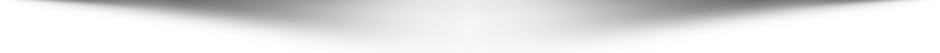# Test Your Knowledge of Mathematics with Our Quiz

Test your math skills with our Mathematics Quiz! This quiz includes 25 questions that cover a variety of mathematical concepts, from basic arithmetic to more advanced topics like algebra and geometry. Each question has four answer choices, so choose the one that you think is correct. At the end of the quiz, you'll be able to see your score and find out how well you did.

Simple Everyday Personal Finance Tips for Everyone - Free

## Mathematics Quiz## All Mathematics Quiz Questions

1. What is the sum of the angles in a triangle?
2. What is the value of pi (π) to two decimal places?
3. What is the formula for the area of a circle?
4. What is the square root of 64?
5. What is the formula for the perimeter of a rectangle?
6. What is the formula for the volume of a sphere?
7. What is the absolute value of -5?
8. What is the formula for the slope of a line?
9. What is the formula for the quadratic equation?
10. What is the Pythagorean theorem?
11. What is the formula for the perimeter of a circle?
12. What is the value of x if 2x + 5 = 11?
13. What is the slope of the line that passes through the points (2, 4) and (6, 8)?
14. What is the value of pi (π) rounded to the nearest hundredth?
15. What is the area of a circle with a radius of 5?
16. What is the value of 6! (6 factorial)?
17. What is the distance between the points (1, 3) and (4, 7)?
18. What is the slope of a vertical line?
19. What is the value of x if 5x + 7 = 32?
20. What is the Pythagorean theorem used for?
21. What is the equation of a line in slope-intercept form?
22. What is the value of the expression 3(x + 2) - 5x when x = 4?
23. What is the area of a rectangle with a length of 8 and a width of 5?
24. What is the formula for the surface area of a cylinder?
25. What is the value of 3 x 52 - 43 + 5 x 2?